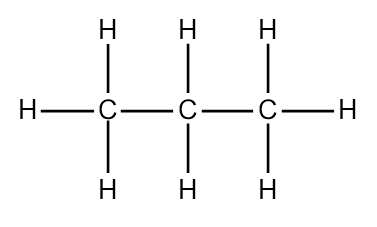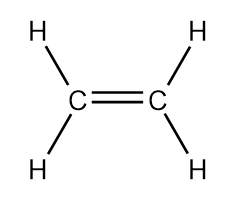# Problem: Two important industrial chemicals, ethene, C2H4, and propene, C3H6, are produced by the steam (or thermal) cracking process:2C3H8(g) ⟶ C2H4(g) + C3H6(g) + CH4(g) + H2(g)For each of the four carbon compounds, do the following:(c) Determine the hybridization of each type of carbon atom.

###### FREE Expert Solution

We are asked to determine the hybridization of each type of carbon atom.

Orbitals that can be used by the central atom:

Subshell                   Total # of orbitals

s             _                 1 orbital

p          _ _ _              3 orbitals

d        _ _ _ _ _          5 orbitals

C3H8(g

C→ Group 4A → 3 x 4 ve = 12 ve

H → Group 1A → 8 x 1 ve = 8 ve

Total = 20 ve1 s orbital + 3 p orbitals = 4 orbitals (4 groups)

s p p p = sp3

The hybridization of each carbon in C3His sp3

C2H4(g

C→ Group 4A → 2 x 4 ve = 8 ve

H → Group 1A → 4 x 1 ve = 4 ve

Total = 12 ve1 s orbital + 2 p orbitals = 3 orbitals (3 groups)

s p p = sp2

The hybridization of each carbon in C2His sp2

88% (194 ratings)###### Problem Details

Two important industrial chemicals, ethene, C2H4, and propene, C3H6, are produced by the steam (or thermal) cracking process:

2C3H8(g) ⟶ C2H4(g) + C3H6(g) + CH4(g) + H2(g)

For each of the four carbon compounds, do the following:

(c) Determine the hybridization of each type of carbon atom.

Frequently Asked Questions

What scientific concept do you need to know in order to solve this problem?

Our tutors have indicated that to solve this problem you will need to apply the Hybridization concept. You can view video lessons to learn Hybridization. Or if you need more Hybridization practice, you can also practice Hybridization practice problems.

What professor is this problem relevant for?

Based on our data, we think this problem is relevant for Professor Fakhreddine's class at TEXAS.

What textbook is this problem found in?

Our data indicates that this problem or a close variation was asked in Chemistry - OpenStax 2015th Edition. You can also practice Chemistry - OpenStax 2015th Edition practice problems.